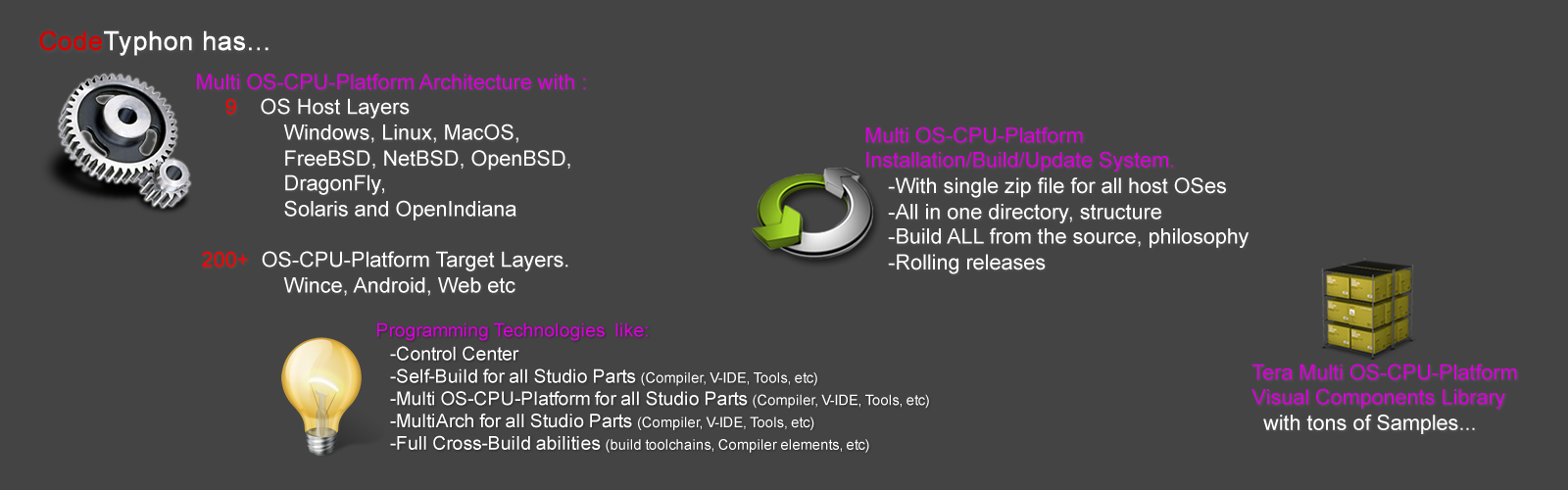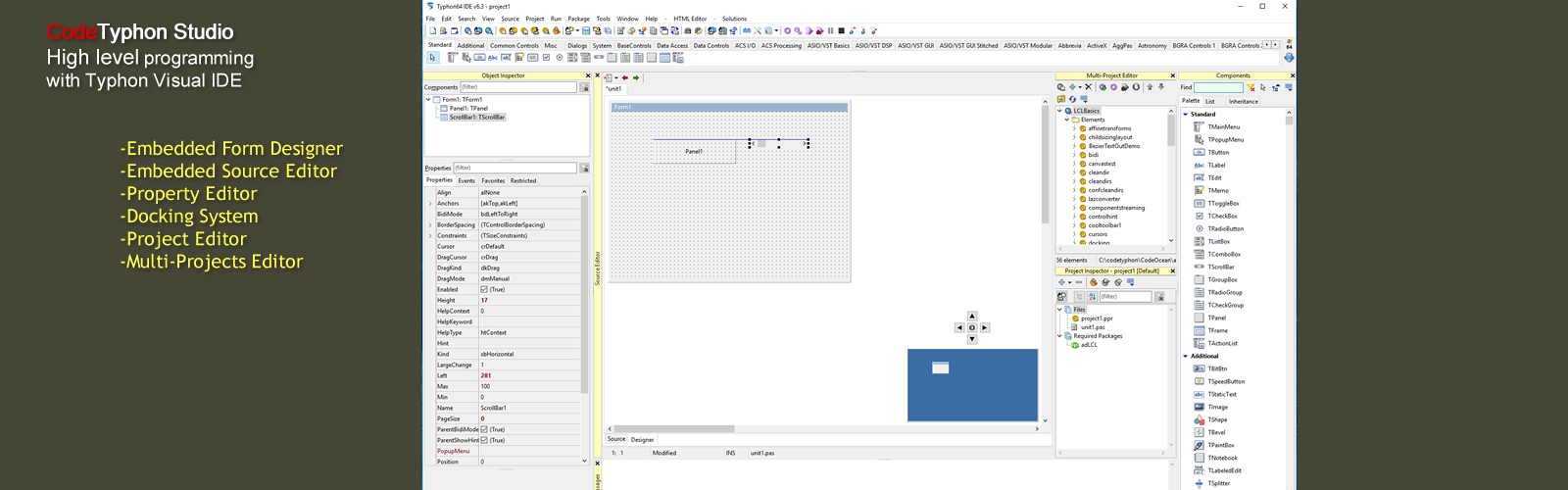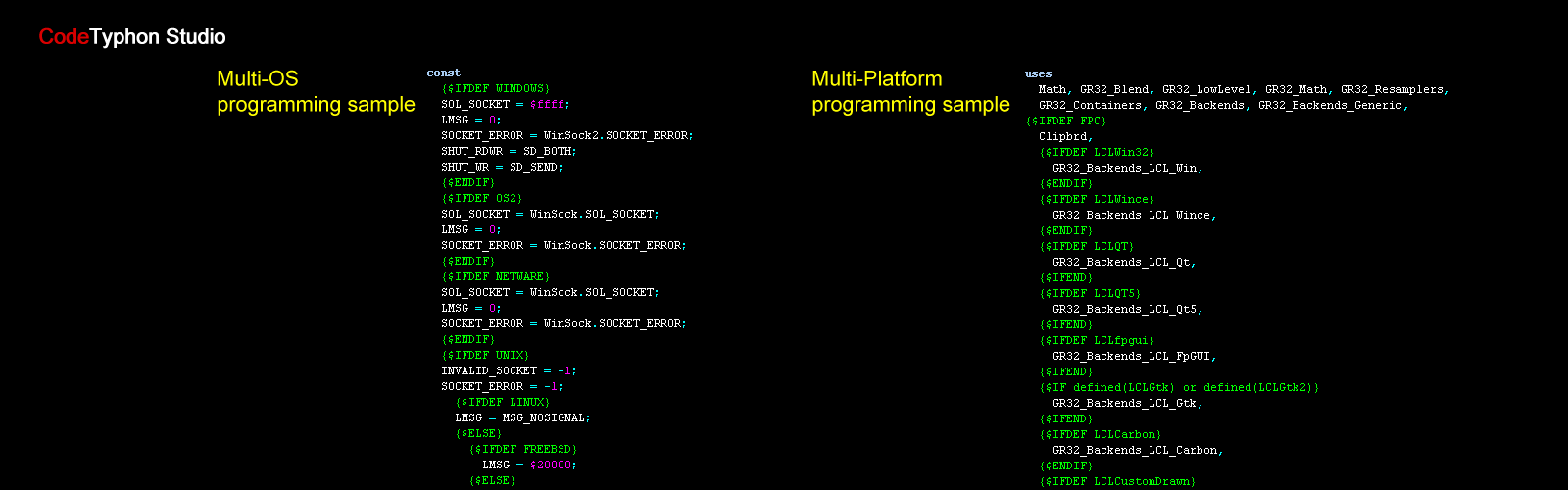## Pascal - Operators

An operator is a symbol that tells the compiler to perform specific mathematical or logical manipulations. Pascal allows the following type of operators:

• Arithmetic operators
• Relational operators
• Boolean operators
• Bit operators
• Set operators
• String operators

Let us discuss the arithmetic, relational, Boolean and bit operators one by one. We will discuss the set operators and string operations later.

## Arithmetic Operators

Following table shows all the arithmetic operators supported by Pascal. Assume variable A holds 10 and variable B holds 20 then:

OperatorDescriptionExample
+ Adds two operands A + B will give 30
- Subtracts second operand from the first A - B will give -10
* Multiply both operands A * B will give 200
div Divide numerator by de-numerator B div A will give 2
mod Modulus Operator and remainder of after an integer division B mod A will give 0

## Relational Operators

Following table shows all the relational operators supported by Pascal. Assume variable A holds 10 and variable B holds 20 then:

OperatorDescriptionExample
= Checks if the value of two operands is equal or not, if yes then condition becomes true. (A = B) is not true.
<> Checks if the value of two operands is equal or not, if values are not equal then condition becomes true. (A <> B) is true.
> Checks if the value of left operand is greater than the value of right operand, if yes then condition becomes true. (A > B) is not true.
< Checks if the value of left operand is less than the value of right operand, if yes then condition becomes true. (A < B) is true.
>= Checks if the value of left operand is greater than or equal to the value of right operand, if yes then condition becomes true. (A >= B) is not true.
<= Checks if the value of left operand is less than or equal to the value of right operand, if yes then condition becomes true. (A <= B) is true.

## Boolean Operators

Following table shows all the Boolean operators supported by Pascal language. All these operators work on Boolean operands and produces Boolean results. Assume variable A holds true and variable B holds false then:

OperatorDescriptionExample
and Called Boolean AND operator. If both the operands are true then condition becomes true. (A and B) is false.
and then It is similar to the AND operator, however, it guarantees the order in which the compiler evaluates the logical expression. Left to right and the right operands are evaluated only when necessary (A and then B) is false.
or Called Boolean OR Operator. If any of the two operands is true then condition becomes true. (A or B) is true.
or else It is similar to Boolean OR, however, it guarantees the order in which the compiler evaluates the logical expression. Left to right and the right operands are evaluated only when necessary (A or else B) is true.
not Called Boolean NOT Operator. Used to reverse the logical state of its operand. If a condition is true then Logical NOT operator will make it false. not (A and B) is true.

## Bit Operators

Bitwise operators work on bits and perform bit by bit operation. All these operators work on integer operands and produces integer results. The truth tables for bitwise and (&), bitwise or (|), and bitwise not (~) are as follows:

pqp & qp | q~p~q
0 0 0 0 1 1
0 1 0 1 1 0
1 1 1 1 0 0
1 0 0 1 0 1

Assume if A = 60; and B = 13; Now in binary format they will be as follows:

A = 0011 1100

B = 0000 1101

-----------------

A&B = 0000 1100

A^B = 0011 0001

~A  = 1100 0011

The Bitwise operators supported by Pascal are listed in the following table. Assume variable A holds 60 and variable B holds 13 then:

OperatorDescriptionExample
& Binary AND Operator copies a bit to the result if it exists in both operands. (A & B) will give 12 which is 0000 1100
| Binary OR Operator copies a bit if it exists in either operand. (A | B) will give 61 which is 0011 1101
! Binary OR Operator copies a bit if it exists in either operand. A ! B) will give 61 which is 0011 1101
~ Binary Ones Complement Operator is unary and has the effect of 'flipping' bits. (~A ) will give -60 which is 1100 0011
<< Binary Left Shift Operator. The left operands value is moved left by the number of bits specified by the right operand. A << 2 will give 240 which is 1111 0000
>> Binary Right Shift Operator. The left operands value is moved right by the number of bits specified by the right operand. A >> 2 will give 15 which is 0000 1111

Please note that different implementations of Pascal differ in bitwise operators. Free Pascal, the compiler we used here, however, supports the following bitwise operators:

OperatorsOperations
not Bitwise NOT
and Bitwise AND
or Bitwise OR
xor Bitwise exclusive OR
shl Bitwise shift left
shr Bitwise shift right
<< Bitwise shift left
>> Bitwise shift right

## Operators Precedence in Pascal

Operator precedence determines the grouping of terms in an expression. This affects how an expression is evaluated. Certain operators have higher precedence than others; for example, the multiplication operator has higher precedence than the addition operator.

For example x = 7 + 3 * 2; Here x is assigned 13, not 20 because operator * has higher precedence than + so it first get multiplied with 3*2 and then adds into 7.

Here operators with the highest precedence appear at the top of the table, those with the lowest appear at the bottom. Within an expression, higher precedence operators will be evaluated first.

OperatorPrecedence
~, not, Highest
*, /, div, mod, and, &
|, !, +, -, or,
=, <>, <, <=, >, >=, in
or else, and then Lowest